# Parabolic SAR Strategy

## Description

Parabolic SAR (Stop and Reversal) strategy is a trading algorithm which role is to predict market trend change and buy / sell an asset in a specific market conditions. It works well in a bull or bear markets. If a market is neither bear nor bull then SAR coefficient does not have to be helpful and SAR coefficient might be ‘belated’.

Values of all the variables defined in the following paragraphs, except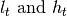, are not defined for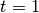.

Lets define by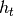(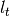) the market high (low) at the end of a day. Moreover,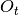is a strategy’s opinion about market trend at the day. It has value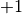if the opinion is uptrend, and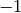otherwise. It will be formally defined later. To define it precisely (by a math expression) we need first define Extreme Point (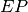), Acceletarion Factor (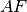) and Stop and Reverse (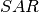) variables.

A variable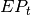, if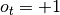, is the highest value of a high from the beginning of the uptrend. Similarly, if market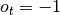, it is the lowest value of low from the beginning of the downtrend. Formally we can defined it as follows: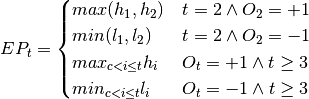where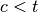is the biggest value for which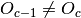. If there is no such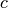(there was no trend change from the time when the strategy starts) then we should setto 1. To sum up: the value ofis the highest high or the lowest low of the current trend.

A variableis a kind of a counter which is set to Acceleration Coefficient when the strategy’s opinion about market trend has changed, it is usually equal to 0.02. Moreover it is increased by Acceleration Coefficient when the new extreme value of EP is found (either high or low depending on the strategy’s opinion about market trend). It is worth to mention that its value cannot exceed Acceleration Limit, by default 0.2. Lets define it formally: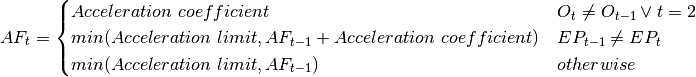Thecoefficient is defined as a function of its previous value, AF and EP. Formally we can defined it as follows: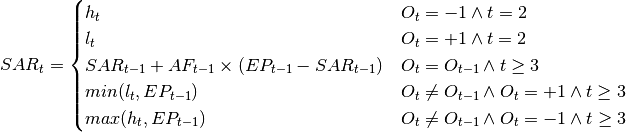Additionally, when SAR indicator is rising it cannot stay above last two intervals’ low price. When the indicator is falling – it cannot stay below maximum of last two intervals’ high price, either. So, if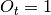and calculatedvalue is bigger than last two periods’ low price (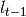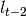), thenvalue is set to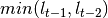. Analogous, if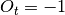and calculatedvalue is smaller than last two periods’ high price (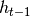,  h_{t-2}), thenvalue is set to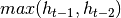.

Finally we can give a formal definition of the. Its definition is the following: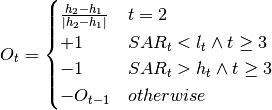As you can see the decision about a actual trend is normally made on the basis of the comparision between the SAR coefficient and the local high (low). At the beginning (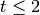) we have to determine whether we have an uptrend or downtrend. In above formula fleeting high prices have been used, but this automatic method can be replaced by a parameter Initial Market Trend basing on the user’s opinion.

## Market Data

• Historical market data

## Parameters

PARAMETER NAME DESCRIPTION ESSENTIAL
Acceleration Coefficient Coefficient of acceleration factor, usually set to 0.02 by default Yes
Acceleration Limit Upper limit of acceleration factor, usually set to 0.2 by default Yes
Initial Market Trend Initial market trend set arbitrary by an user No

## Conditions

### Open position

Side Buy or Sell
Amount One asset per order
Price Last market price
Type Price limit

Consider day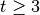. If strategy does not have an open position and:

•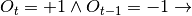algorithm opens long position
•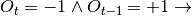algorithm opens short position

### Close position

Consider day. If strategy does have an open position and:

•algorithm closes short position
•algorithm closes long position

### Termination

There is no strategy termination condition.

### Time frame

Strategy is designed for long-term trading, usually for whole weeks.